﻿ Analytical investigation of the Laminar Viscous Flow in a Semi-Porous Channel in the Presence of a Uniform Magnetic Field

### Analytical investigation of the Laminar Viscous Flow in a Semi-Porous Channel in the Presence of a U...

A. Majidian, M. Fakour, A. VahabzadehOPEN ACCESSPEER-REVIEWED

## Analytical investigation of the Laminar Viscous Flow in a Semi-Porous Channel in the Presence of a Uniform Magnetic Field

A. Majidian1, M. Fakour1,, A. Vahabzadeh1

1Department of Mechanical Engineering, Sari Branch, Islamic Azad University, Sari, Iran

### Abstract

In this paper, the laminar fluid flow in a semi-porous channel in the presence of transverse magnetic field is investigated. The homotopy perturbation method (HPM) is employed to compute an approximation to the solution of the system of nonlinear differential equations governing the problem. It has been attempted to exhibit the reliability and performance of the homotopy perturbation method (HPM) in comparison with the numerical method (Richardson extrapolation) in solving this problem. The influence of the two dimensionless numbers: the Hartmann number and Reynolds number on non-dimensional velocity profile are considered. The results indicate that velocity boundary layer thickness decrease with increase of Reynolds number and it increases as Hartmann number increases.

### At a glance: Figures

123
Prev Next

• Majidian, A., M. Fakour, and A. Vahabzadeh. "Analytical investigation of the Laminar Viscous Flow in a Semi-Porous Channel in the Presence of a Uniform Magnetic Field." International Journal of Partial Differential Equations and Applications 2.4 (2014): 79-85.
• Majidian, A. , Fakour, M. , & Vahabzadeh, A. (2014). Analytical investigation of the Laminar Viscous Flow in a Semi-Porous Channel in the Presence of a Uniform Magnetic Field. International Journal of Partial Differential Equations and Applications, 2(4), 79-85.
• Majidian, A., M. Fakour, and A. Vahabzadeh. "Analytical investigation of the Laminar Viscous Flow in a Semi-Porous Channel in the Presence of a Uniform Magnetic Field." International Journal of Partial Differential Equations and Applications 2, no. 4 (2014): 79-85.

 Import into BibTeX Import into EndNote Import into RefMan Import into RefWorks

### 1. Introduction

The flow problem in porous tubes or channels received much attention in recent years because of its various applications in biomedical engineering, for example, in the dialysis of blood in artificial kidney , in the flow of blood in the capillaries , in the flow in blood oxygenators , as well as in many other engineering areas such as the design of filters , in transpiration cooling boundary layer control  and gaseous diffusion . In 1953, Berman  described an exact solution of the Navier-Stokes equation for steady two dimensional laminar flow of a viscous, incompressible fluid in a channel with parallel, rigid, porous walls driven by uniform, steady suction or injection at the walls. This mass transfer is paramount in some industrial processes. In the pastyears, many studies have been published on problems related to the influence of an induction on the dynamic and thermodynamic fields, Osterle and Young  and Umavathi  considered the natural convection between two parallel plates, Askovic et al.  or Askovic  presented some developments of three dimensional MHD problems in case of non-stationary flows or flows induced by accelerated or deformable bodies. More recently, Chandran and Sacheti  analyzed the effects of a magnetic field on the thermodynamic flow past a continuously moving porous plate.

Nomenclature

HPM: Homotopy perturbation method

NUM: Numerical method

P: Pressure

q: Mass transfer parameter

Re: Reynolds number

U: Dimensionless velocity in the x direction

V: Dimensionless velocity in the y direction

h: Suspension height

Ha: Hartman number

Lx: Length of the slider

u0: x velocity of the pad

u: Dimensionless x −component velocity

v: Dimensionless y −component velocity

u*: Velocity component in the x direction

v*: Velocity component in the y direction

x: Dimensionless horizontal coordinate

y: Dimensionless vertical coordinate

x*: Distance in the x direction parallel to the plates

y*: Distance in the y direction parallel to the plates

Greek Symbols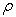: Fluid density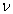: Kinematic viscosity: Electrical conductivity: Aspect ratio h / Lx

Simultaneously, Chamkha [13, 14] has detailed the influence of a magnetic field on an electrically conducting fluid in the neighborhood of a cone, a wedge or near a stagnation point. More recently, Desseaux  analyzed the influence of a magnetic field over a laminar viscous flow in a semi-porous channel. All these problems and phenomena are modeled by ordinary or partial differential equations. d In recent years, much attention has been devoted to the newly developed methods to construct an analytical solution of equation. In this paper, the basic idea of the HPM is introduced and then the coupled ordinary non-linear differential equations of the laminar viscous flow in a semi-porous channel are solved through the HPM. The effects of the Hartmann number and Reynolds number on velocity profile are considered.

### 2. Describe Problem and Mathematical Formulation

Consider the laminar two-dimensional stationary flow of an electrically conducting incompressible viscous fluid in a semi-porous channel made by a long rectangular plate of length Lx in uniform translation in x* direction and an infinite porous plate. The distance between the two plates is h. The physical fluid properties (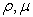) are constant. We observe a normal velocity q at the porous wall. A uniform magnetic field B is assumed to be applied towards direction y* (Figure 1) .

In the case of a short circuit to neglect the electrical field and perturbations to the basic normal field and without any gravity forces, according to Osterle and Young , Umavathi , the governing equations are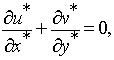(1)(2)(3)
Figure 1. Schematic of the problem (fluid in a porous media between parallel plates and magnetic field)

The appropriate boundary conditions for the velocity are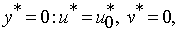(4)(5)

Calculating a mean velocity U by the relation(6)

We consider the following transformations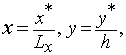(7)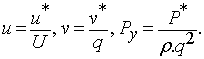(8)

Then, we can consider two dimensionless numbers: the Hartman number Ha for the description of the magnetic forces  and the Reynolds number Re for the dynamic forces(9)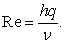(10)

Introducing Eqs. (6) and (10) in Eqs. (1) and (3) leads to the dimensionless equations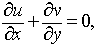(11)(12)(13)

Quantityis defined as the ratio between distance h and a characteristic length Lx of the slider. This ratio is normally small. Berman’s similarity transformation is used to be free from the aspect ratio ε: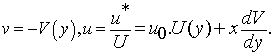(14)

Introducing Eq. (14) in the second momentum equation (13) shows that quantity ∂Py / y does not depend on the longitudinal variable x. With the first momentum equation, we see also that ∂2Py / 2x is independent of x. We omit asterisks for simplicity. Then a separation of variables leads to(15)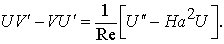(16)

The right-hand side of Eq. (15) is constant. So, we derive this equation with respect to y. This gives(17)

where primes denote differentiation with respect to y and asterisks have been omitted for simplicity. The dynamic boundary conditions become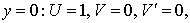(18)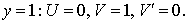(19)

### 3. Describe Homotopy Perturbation Method and Applied to the Problem

3.1. Describe Homotopy Perturbation Method

Consider the following function:(20)

with the boundary condition of: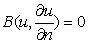(21)

where A (u) is defined as follows:(22)

Homotopy-perturbation structure is shown as:(23)

or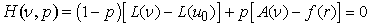(24)

where(25)

Obviously, considering Eqs. (23) and (24), we have: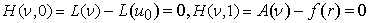(26)

where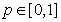is an embedding parameter and u0 is the first approximation that satisfies the boundary condition. The process of the changes in p from zero to unity is that of v(r, p) changing from u0 to ur. We consider v as:(27)

and the best approximation is:(28)

the above convergence is discussed in [16, 19].

3.2. The HPM Applied to the Problem

A homotopy perturbation method can be constructed as follows: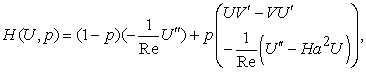(29)(30)

One can now try to obtain a solution of Eqs. (29, 30) in the form of:(31)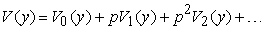(32)

where vi(Y), i=1,2,3,… are functions yet to be determined. According to Eqs. (29,30) the initial approximation to satisfy boundary condition is: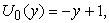(33)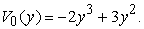(34)

Substituting Eqs.(31, 32) into Eqs. (29, 30) yields: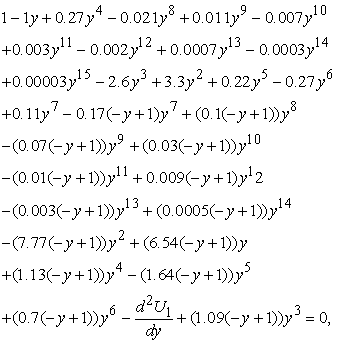(35)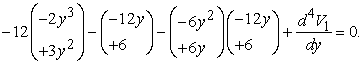(36)

The solutions of Eqs. (35, 36) may be written as follow: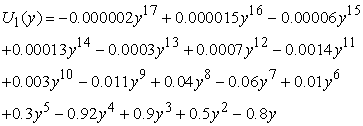(37)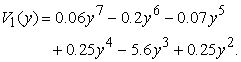(38)

In the same manner, the rest of components were obtained by using the Maple package. According to the HPM, we can conclude: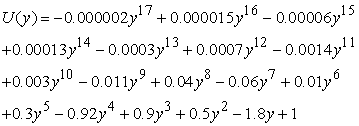(39)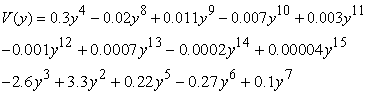(40)

and so on. In the same manner the rest of the components of the iteration formula can be obtained.

### 4. Results and discussion

In this paper, Similarity Berman’s transformation is employed to convert the governing partial differential equations of a steady laminar flow of an electrically conducting fluid in a two dimensional channel. The HPM was applied successfully to find the analytical solution of resulting ordinary differential equation.The numerical solution is performed using the algebra package Maple 16.0, to solve the present case. The package uses a Richardson extrapolation procedure for solving nonlinear boundary value (B-V) problem. Table 1 shows the comparison between numerical solution and HPM method for U and V. This is notable that the value of U and V are obtained by using HPM with 3 times but the values of U and Vare obtained by using DTM with 20 times . Also the values obtained by HPM method are closer to the values obtained by numerically method. So we can conclude that the HPM method is a powerful analytical method to solve such this problems.

#### Table 1. The results of the HPM and numerical methods for V ( y), U(y) for Ha = 1 and Re = 1Download asPowerPoint Slide

Veiw figureView current table in a new window

Figure 6. Effect of Hartman number (Ha) on dimensionless velocities, a) V(y), Re = 1, b) U(y), Re = 1, c) V(y), Re = 5 and d) U(y), Re = 5
Figure 7. Effect of Reynolds number (Re) on dimensionless velocities, a) V(y), Ha = 1, b) U(y), Ha = 1, c) V(y), Ha = 10 and d) U(y), Ha = 10

Figure 2 and Figure 3 show comparison between the numerical solution and the HPM solution for U( y) and V( y), when Re =1 and Ha = 1. Figure 4 and Figure 5 illustrate the accuracy of the HPM solution compare to numerical solution when Re =1 and Ha =0.

Effect of Hartman number (Ha) on dimensionless velocities is shown in Figure 6. Generally, when the magnetic field is imposed on the enclosure, the velocity field suppressed owing to the retarding effect of the Lorenz force. For example Figure 6(b) shows that as Hartmann number increases from 0 to 5, velocity boundary layer thickness decreases. For low Reynolds number, as Hartmann number increases V(y) decreases for y > ym but opposite trend is observed for y < ym, ym is a meeting point that all curves joint together at this point. When Reynolds number increases this meeting point shifts to the solid wall and it can be seen that V(y) decreases with increase of Hartmann number.

Effect of Reynolds number (Re) on dimensionless velocities is shown in Figure 7. As seen in Figure 7, when Ha number is small the effect of Re number is more sensible and increasing the Re number, makes a decrease in velocity profiles also it is worth to mention that the Reynolds number indicates the relative significance of the inertia effect compared to the viscous effect. Thus, velocity boundary layer thickness on lower plate decreases as Re increases and in turn increasing Re leads to an increase in the magnitude of the skin friction coefficient.

### 5. Conclusion

In this paper, Homotopy perturbation method is used to solve the problem of laminar fluid flow in a semi-porous channel in the presence of uniform magnetic field. In this case study, the Berman’s similarity transformation has been used to reduce the governing differential equations into a set of coupled ordinary non-linear differential equations. In most cases, these problems do not admit analytical solution, so these equations should be solved using special techniques.It has been attempted to exhibit the reliability and performance of the Homotopy perturbation method (HPM) in comparison with the numerical method (Richardson extrapolation) in solving this problem. The results indicate that velocity boundary layer thickness decrease with increase of Reynolds number and it increases as Hartmann number increases.

### References

  V. Wernert, O. Schäf, H. Ghobarkar and R. Denoyel, Adsorption properties of zeolites for artificial kidney applications, Microporous and Mesoporous Materials, 83 1-3 (2005), 101-113.In article  A. Jafari, P. Zamankhan, S.M. Mousavi and P. Kolari, Numerical investigation of blood flow Part II: In capillaries, Communications in Nonlinear Science and Numerical Simulation, 14 (2009), 1396-1402.In article CrossRef  A.R. Goerke, J. Leung and S. R. Wickramasinghe, Mass and momentum transfer in blood oxygenators, Chemical Engineering Science, 57 (2002), 2035-2046.In article CrossRef  S.S. Mneina and G.O. Martens, Linear phase matched filter design with causal real symmetric impulse response, AEU-International Journal of Electronics and Communications, 63 (2009), 83-91.In article CrossRef  Y. H. Andoh and B. Lips, Prediction of porous walls thermal protection by effusion or transpiration cooling: An analytical approach, Applied Thermal Engineering, 23 (2003), 1947-1958.In article CrossRef  A. Runstedtler, On the modified Stefan–Maxwell equation for isothermal multicomponent gaseous diffusion, Chemical Engineering Science, 61 (2006), 5021-5029.In article CrossRef  A.S. Berman, Journal of Applied Physics, 24 (1953), 1232.In article CrossRef  J.F. Osterle and F.J. Young, Journal of Fluid Mechanics, 1 (1961), 512.In article CrossRef  J.C. Umavathi, International Journal of Non-Linear Mechanics, 31 (1996), 371.In article CrossRef  R. Askovic, P. Florent, C. Tournier and R. Roum, Science Technology, 35 (1990), 295.In article  R. Askovic, International Conference on Fluid and Thermodynamics- Indonesia, volume 1 (1994), 263.In article  P. Chandran, N.C. Sacheti and A.K. Singh, Internationa Communications of Heat and Mass Transfer, 23 (1996), 889.In article CrossRef  A.J. Chamkha, International Communications of Heat and Mass Transfer, 23 (1996), 875.In article CrossRef  A.J. Chamkha, International Communications of Heat and Mass Transfer, 25, (1998), 269.In article CrossRef  A. Desseaux, Influence of a magnetic field over a laminar viscous flow in a semi-porous channel, International journal of Engineering Science, 37 (1999), 94.In article CrossRef  M. Fakour, A. Vahabzadeh, D.D. Ganji,Scrutiny of mixed convection flow of a nanofluid in a vertical channel, International journal of Case Studies in Thermal Engineering, 4 (2014), 15-23.In article CrossRef  M. Fakour, A. Vahabzadeh, D.D. Ganji, I.Rahimi Petroudi, “Analytical accuracy of the one dimensional heat transfer in geometry with logarithmic various surfaces,” Cent. Eur. J. Eng., vol. 4, pp. 341-355, 2014.In article CrossRef  Ganji, D.D. and Sadighi, A. ‘‘Application of homotopy-perturbation and variational iteration methods to nonlinear heat transfer and porous media equations’’, Journal of Computational and Applied Mathematics, 207, pp. 24-34 (2007).In article CrossRef  M. Fakour, D.D. Ganji, A. Vahabzadeh, S.H.H. Kachapi, Accuracy of VIM, HPM and ADM in Solving Nonlinear Equations for the Steady Three-Dimensional Flow of a Walter’s B Fluid in Vertical Channel, Walailak Journal of Science and Technology, 11 (2014), 7, 593-609.In article  M. M. Rashidi and S. M. Sadri,Int. J. Contemp. Math. Sciences, Vol. 5, 2010, no. 15, 711-720.In article